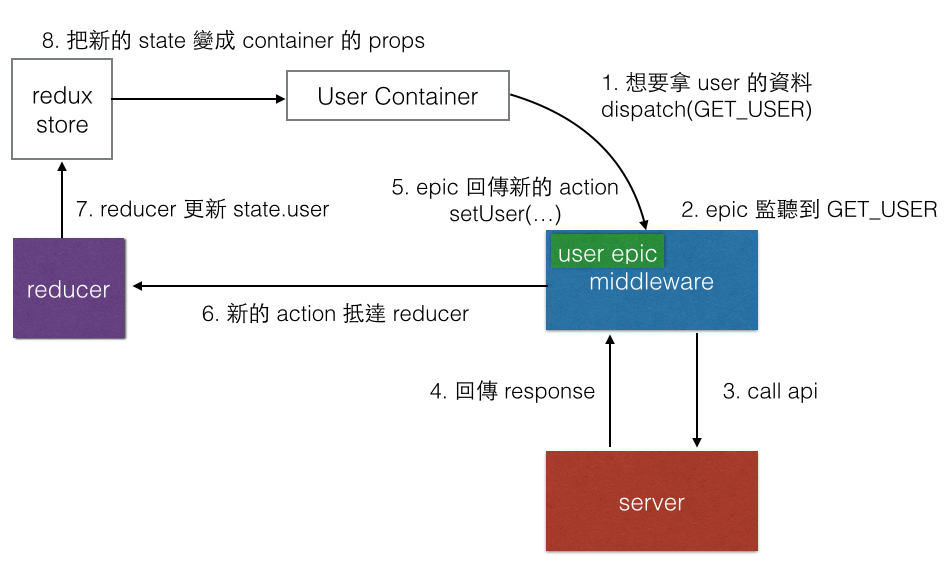# 程式基礎能力測試

``````const source = [1, 5, 9, 3, 'hi', 'tb', 456, '11', 'yoyoyo'];
let total = 0;

for (let i = 0; i < source.length; i++) {
let num = parseInt(source[i], 10);
if (!isNaN(num)) {
total += num * 2;
}
}
``````

``````const source = [1, 5, 9, 3, 'hi', 'tb', 456, '11', 'yoyoyo'];

let total = source
.map(x => parseInt(x, 10))
.filter(x => !isNaN(x))
.map(x => x * 2)
.reduce((total, value) => total + value )
``````

Imperative 是命令機器去做事情（how），這樣不管你想要的是什麼（what），都會按照你的命令實現；Declarative 是告訴機器你想要的是什麼（what），讓機器想出如何去做（how）

``````SELECT * from dogs INNER JOIN owners WHERE dogs.owner_id = owners.id
``````

``````//dogs = [{name: 'Fido', owner_id: 1}, {...}, ... ]
//owners = [{id: 1, name: 'Bob'}, {...}, ...]

var dogsWithOwners = []
var dog, owner

for(var di=0; di < dogs.length; di++) {
dog = dogs[di]
for(var oi=0; oi < owners.length; oi++) {
owner = owners[oi]
if (owner && dog.owner_id == owner.id) {
dogsWithOwners.push({
dog: dog,
owner: owner
})
}
}
}
``````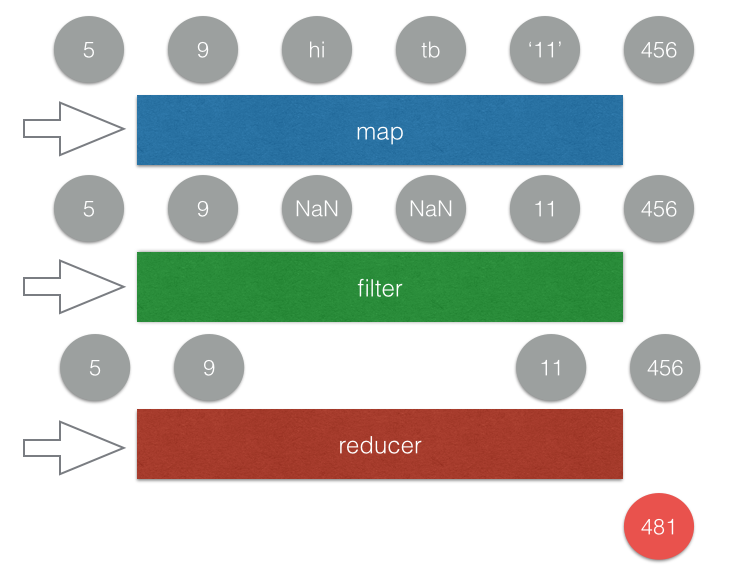# Reactive Programming

``````window.addEventListener('click', function(){
console.log('click!');
})
``````

# 正式進入 RxJS

ReactiveX is a combination of the best ideas from
the Observer pattern, the Iterator pattern, and functional programming

``````Rx.Observable.fromEvent(window, 'click')
.subscribe(e => {
console.log('click~');
})
``````

Observable 就是一個可被觀察的對象，這個對象可以是任何東西（例如說上述例子就是 window 的 click 事件），當有新資料的時候（例如說新的點擊事件），你就可以接收到這個新資料的資訊並且做出反應。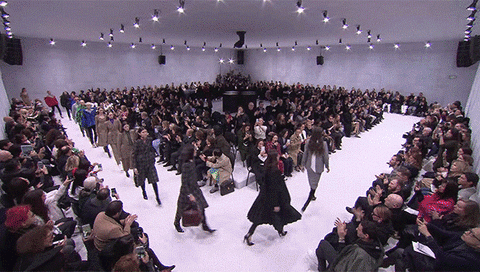（圖片取自 giphy

# 我理解資料流了，然後呢？

``````Rx.Observable.fromEvent(window, 'click')
.map(e => e.target)
.subscribe(value => {
console.log('click: ', value)
})
``````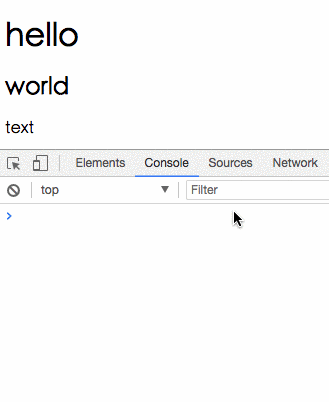``````Rx.Observable.fromEvent(window, 'click')
.map(e => 1)
.scan((total, now) => total + now)
.subscribe(value => {
document.querySelector('#counter').innerText = value;
})
``````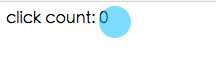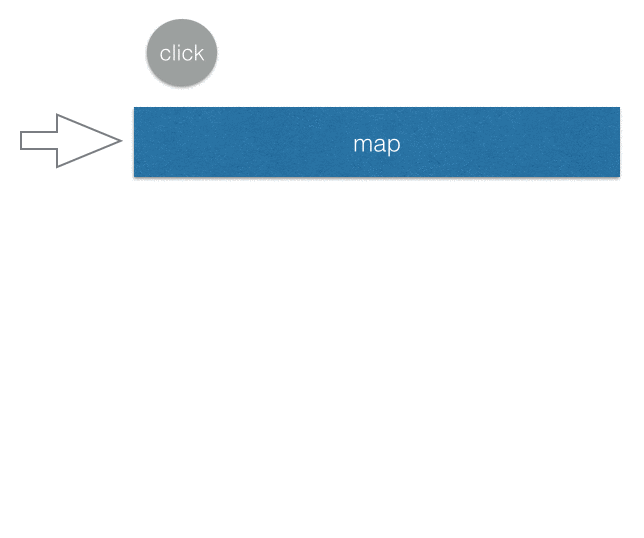# 威力無窮的組合技

Observable 跟陣列的差別就在於多了一個維度：時間。

Observable 是「時間序列上的一連串資料事件」，就像我前面講的一樣，可以看成是一個一直會有新資料進來的陣列。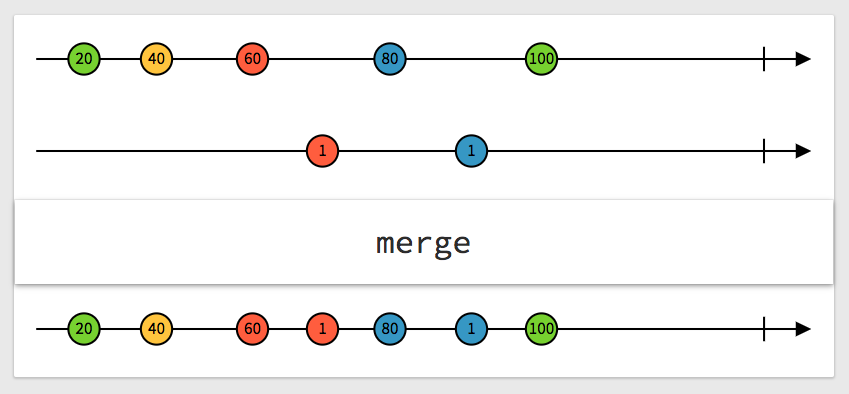（取自：http://rxmarbles.com/#merge）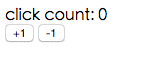``````Rx.Observable.fromEvent(document.querySelector('input[name=plus]'), 'click')
.mapTo(1)
.merge(
Rx.Observable.fromEvent(document.querySelector('input[name=minus]'), 'click')
.mapTo(-1)
)
.scan((total, now) => total + now)
.subscribe(value => {
document.querySelector('#counter').innerText = value;
})
``````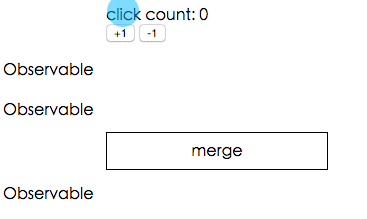``````var total = 0;
total++;
document.querySelector('#counter').innerText = total;
})

total--;
document.querySelector('#counter').innerText = total;
})
``````

# 組合技中的組合技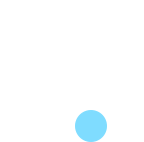``````var canvas = document.getElementById('canvas');
var ctx = canvas.getContext('2d');
ctx.beginPath(); // 開始畫畫

function draw(e){
ctx.lineTo(e.clientX,e.clientY); // 移到滑鼠在的位置
ctx.stroke(); // 畫畫
}

// 按下去滑鼠才開始偵測 mousemove 事件
ctx.moveTo(e.clientX, e.clientY); // 每次按下的時候必須要先把繪圖的點移到那邊，否則會受上次畫的位置影響
})

// 放開滑鼠就停止偵測
canvas.removeEventListener('mousemove', draw);
})
``````

``````Rx.Observable.fromEvent(canvas, 'mousedown')
.subscribe(e => {
console.log('mousedown');
})
``````

``````Rx.Observable.fromEvent(canvas, 'mousedown')
.mapTo(
Rx.Observable.fromEvent(canvas, 'mousemove')
)
.subscribe(value => {
console.log('value: ', value);
})
``````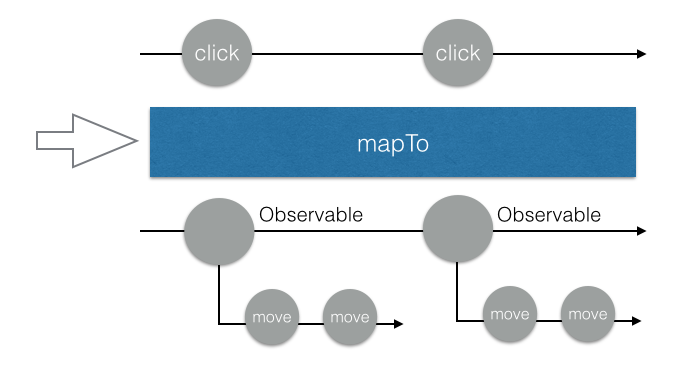``````Rx.Observable.fromEvent(canvas, 'mousedown')
.flatMap(e => Rx.Observable.fromEvent(canvas, 'mousemove'))
.subscribe(e => {
console.log(e);
})
``````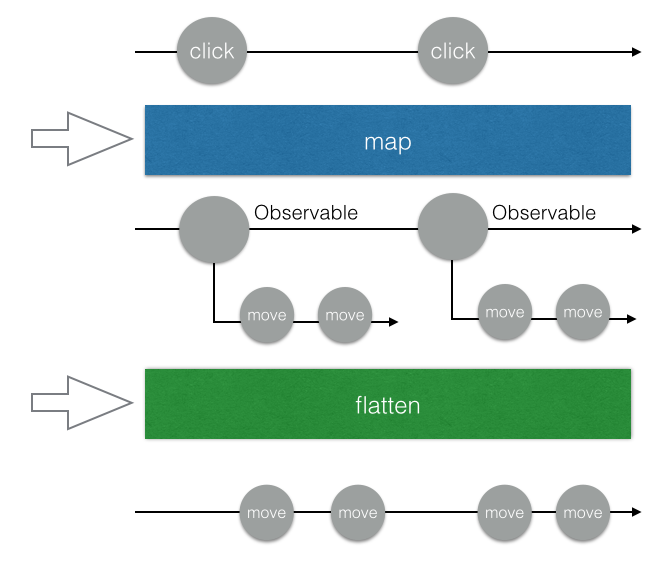``````Rx.Observable.fromEvent(canvas, 'mousedown')
.flatMap(e => Rx.Observable.fromEvent(canvas, 'mousemove'))
.takeUntil(Rx.Observable.fromEvent(canvas, 'mouseup'))
.subscribe(e => {
draw(e);
})
``````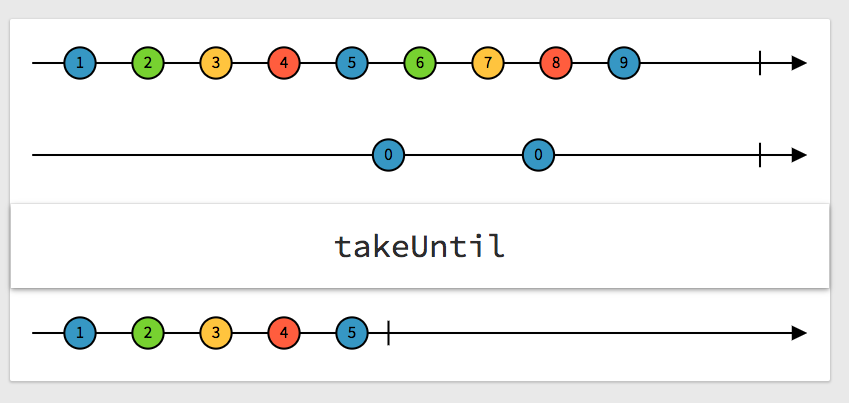``````Rx.Observable.fromEvent(canvas, 'mousedown')
.flatMap(e => Rx.Observable.fromEvent(canvas, 'mousemove')
.takeUntil(Rx.Observable.fromEvent(canvas, 'mouseup'))
)
.subscribe(e => {
draw(e);
})
``````

``````Rx.Observable.fromEvent(canvas, 'mousedown')
.do(e => {
ctx.moveTo(e.clientX, e.clientY)
})
.flatMap(e => Rx.Observable.fromEvent(canvas, 'mousemove')
.takeUntil(Rx.Observable.fromEvent(canvas, 'mouseup'))
)
.subscribe(e => {
draw(e);
})
``````

# 喝口水休息一下，下半場要開始了

1. 一個資料流可以經過一系列轉換，變成另一個資料流
2. 這些轉換基本上都跟陣列有的差不多，像是`map``filter``flatten`等等
3. 你可以合併多個 Observable，也可以把二維的 Observable 壓平

``````function sendRequest () {
return fetch('https://jsonplaceholder.typicode.com/posts/1').then(res => res.json())
}

Rx.Observable.fromEvent(document.querySelector('input[name=send]'), 'click')
.flatMap(e => Rx.Observable.fromPromise(sendRequest()))
.subscribe(value => {
console.log(value)
})
````````````function searchWikipedia (term) {
return \$.ajax({
url: 'http://en.wikipedia.org/w/api.php',
dataType: 'jsonp',
data: {
action: 'opensearch',
format: 'json',
search: term
}
}).promise();
}

function renderList (list) {
\$('.auto-complete__list').empty();
\$('.auto-complete__list').append(list.map(item => '<li>' + item + '</li>'))
}
``````

``````[你輸入的關鍵字, 關鍵字清單, 每個關鍵字的介紹, 每個關鍵字的連結]

// 範例：
[
"dd",
["Dd", "DDR3 SDRAM", "DD tank"],
["", "Double data rate type three SDRAM (DDR3 SDRAM)", "DD or Duplex Drive tanks"],
[https://en.wikipedia.org/wiki/Dd", "https://en.wikipedia.org/wiki/DDR3_SDRAM", "...略"]
]
``````

``````document.querySelector('.auto-complete input').addEventListener('input', (e) => {
searchWikipedia(e.target.value).then((data) => {
renderList(data)
})
})
``````

``````var timer = null;
if (timer) {
clearTimeout(timer);
}
timer = setTimeout(() => {
searchWikipedia(e.target.value).then((data) => {
renderList(data)
})
}, 250)
})
``````

``````var timer = null;
if (timer) {
clearTimeout(timer);
}
timer = setTimeout(() => {
searchWikipedia(e.target.value).then((data) => {
if (data === document.querySelector('.auto-complete input').value) {
renderList(data)
}
})
}, 250)
})
``````

``````Rx.Observable
.fromEvent(document.querySelector('.auto-complete input'), 'input')
.map(e => e.target.value)
.flatMap(value => {
return Rx.Observable.from(searchWikipedia(value)).map(res => res)
})
.subscribe(value => {
renderList(value);
})
``````

RxJS 已經幫你實作好了，所以你只要加上`.debounceTime(250)`就好了，就是這麼簡單。

``````Rx.Observable
.fromEvent(document.querySelector('.auto-complete input'), 'input')
.debounceTime(250)
.map(e => e.target.value)
.flatMap(value => {
return Rx.Observable.from(searchWikipedia(value)).map(res => res)
})
.subscribe(value => {
renderList(value);
})
``````

Observable 有一個不同的解法，我來解釋給大家聽聽。

`switchMap`的差別在於，他永遠只會處理最後一個 Observable。拿我們的例子來說，假設第一個 request 還沒回來的時候，第二個 request 就發出去了，那我們的 Observable 就只會處理第二個 request，而不管第一個。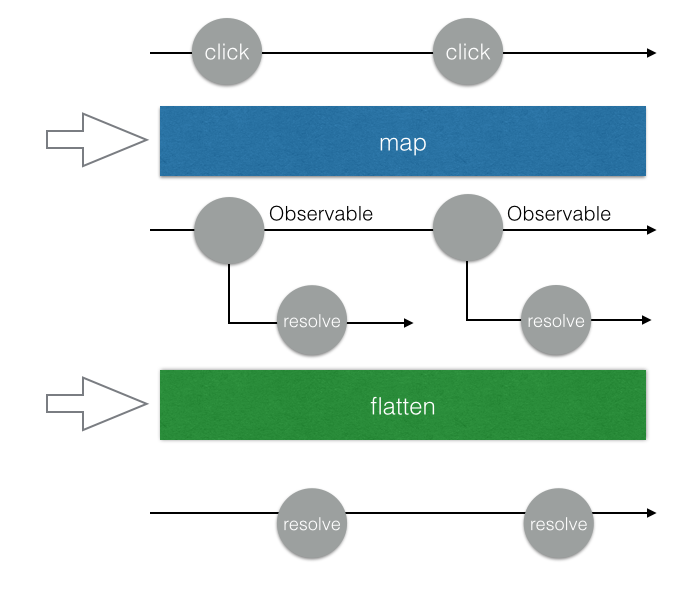`switchMap`只會處理最後一個：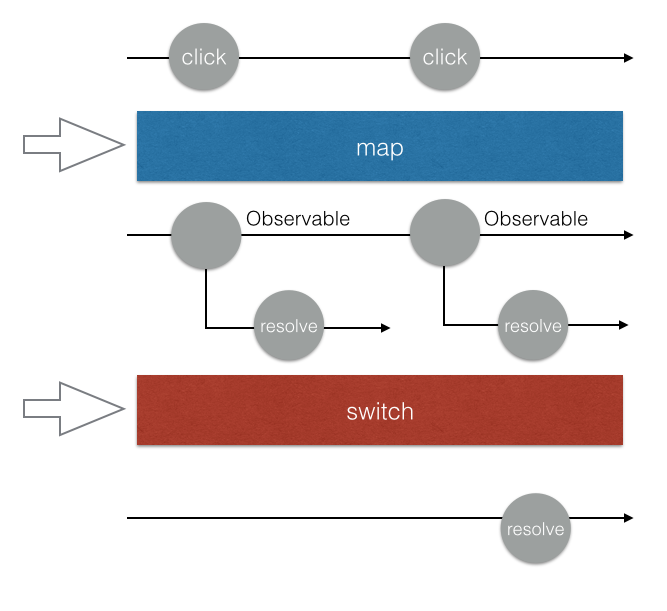``````Rx.Observable
.fromEvent(document.querySelector('.auto-complete input'), 'input')
.debounceTime(250)
.map(e => e.target.value)
.switchMap(value => {
return Rx.Observable.from(searchWikipedia(value)).map(res => res)
})
.subscribe(value => {
renderList(value);
})
``````

``````Rx.Observable
.fromEvent(document.querySelector('.auto-complete input'), 'input')
.debounceTime(250)
.map(e => e.target.value)
.switchMap(value => {
return value.length < 1 ? Rx.Observable.of([]) : Rx.Observable.from(searchWikipedia(value)).map(res => res)
})
.subscribe(value => {
renderList(value);
})
``````

``````Rx.Observable
.fromEvent(document.querySelector('.auto-complete__list'), 'click')
.filter(e => e.target.matches('li'))
.map(e => e.target.innerHTML)
.subscribe(value => {
document.querySelector('.auto-complete input').value = value;
renderList([])
})
``````

# React + Redux 的非同步解決方案：redux-observable

React + Redux 這一套非常常見的組合，一直都有一個問題存在，那就是沒有規範非同步行為（例如說 API）到底應該怎麼處理。而開源社群也有許多不同的解決方案，例如說 redux-thunk、redux-promise、redux-saga 等等。

``````// 範例而已
Rx.Observable.from(actionStreams)
.subscribe(action => {
})
``````

``````Rx.Observable.from(actionStreams)
.filter(action => action.type === 'GET_USER_INFO')
.switchMap(
)
.subscribe(userInfo => {
dispatch({
type: 'SET_USER_INFO',
})
})
``````

action in, action out

`redux-observable` 是一個 middleware，你可以在裡面加上很多`epic`，每一個`epic`就是一個 Observable，你可以監聽某一個指定的 action，做一些處理，再轉成另外一個 action。

``````import Actions from './actions/user';
import ActionTypes from './actionTypes/user'

const getUserEpic = action\$ =>
action\$.ofType(actionTypes.GET_USER)
.switchMap(
).map(userInfo => Actions.setUsers(userInfo))
``````